# [原创图文] C语言学习第九天 数组即练习（上）

19主题 3精华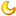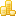学币
229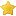荣耀
0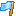rank
0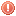违规
0发表于 2020-12-18 15:05:17 | 显示全部楼层 |阅读模式
 本帖最后由 鸦领主 于 2020-12-23 15:22 编辑 （之所以定义一个数组是因为要同时定义多个同类型的变量，节省内存） 1.数组： 数组是一组具有相同数据类型的有序变量的集合，再内存中表现为一块连续的存储区域。 a）数组定义:普通变量定义后加[常量] 如：类型标识符 数组名[常量表达式]        char           s        而后的scanf_s("%s",s,sizeof(s)) s可以用&也可以不用 因为s是char数组，s本身指向这个数组首地址。 s里保存的本身就是个地址。&是取地址，所以可以用也可以不用 b）数组初始化：用{}给数组赋予初值 初始化元素的个数可以不全（用1个到总数个常量和变量） 未初始化全，把其余的归零 数组清零:char s={0} 不指定个数 int s[]={2,55,36,44} c)求数组空间： sizeof(数组)也可以说是sizeof(元素)*元素的个数 _countof(数组)用到#include 2.定义一个字符串数组，循环打印出每个数组的内容，一直到结尾符'\0'结束（\0占一个位置） #include int main() {     char c;//定义一个20空间的字符串数组     scanf_s("%s", &c,20);    //指定20个长度给scanf_s使用     //或则使用sizeof(s)更方便，上面变化下面不用改动     int i = 0;         while (c[i]!='\0')//到结尾符结束循环或者可以写成c[i]!=0         {             printf("c[%d]:%c=%d 地址:0x%x\n",i,c,c,&c);             i++;         }     return 0; } 数组3.定义一个字符串数组，得出你输入的字符串的长度 #include int main() {     char c;     scanf_s("%s", &c,sizeof(c));        int i = 0;     while (c[i][i])//这里可以简化成这个样子     i++;       printf("你输入了%d个字符串", i);     return 0; } 数组4.定义一个字符串数组,将输入的字符串反序输出(①反一个输出一个，②全部反序再输出) 汉字反序的话就会变成另一个汉字，不再是原来的汉字 ① #include int main() {     char c;     scanf_s("%s", &c,sizeof(c));        int i = 0;     while (c[i])         i++;     while (i > 0)         printf("%c",c[--i]);              return 0; }② #include int main() {     char c;     scanf_s("%s", &c,sizeof(c));        int i = 0;     while (c[i])         i++;     int n=0;     while (--i>n)     {                                                                int t = c[n]; //把n的值先给t                           c[n] = c[i];//再把i的值给n                          c[i]= t;//最后把n也就是t的值给i               n++;     }     printf("%s", c);     return 0; } } 123456789 i=9，n=1 t=n(t=1) n=i(n=9)此时是 923456789 i=t(i=1)此时是 923456781 经过循环i--，n++ i=8，n=2 t=n(t=2) n=i(n=8)此时是 983456789 i=t(i=2)此时是 983456721 .... 数组5.定义一个整数数组，输入几个数字用00做结尾符，求出他们的总和和平局值 #include int main() {     int i;                                int n = -1;                               do                                         {                                                //没有办法像字符串一样一次性输入进去         scanf_s("%d", &i[++n]);       //做一个循环，比如 40 30 55用00做结尾符     } while (i[n] != 00);                    //第一次把40放入i,再次循环把30放入i.....     float f = 0;     n = 0;     while (i[n]!=00)     {         f = f + i[n++];     }     printf("总和是%0.1f平局值是:%0.2f", f, f / n);     return 0; } 数组6.定义一个整数数组，随便输入几个数字，实现冒泡排序 #include #include int main() {     int i;     int y = 0;     printf("请随机输入%d个数字进行排序:\n", _countof(i));     while (y < _countof(i))     {         scanf_s("%d", &i[y++]);     } //===================中间为核心算法     y = 0;     while (y< _countof(i)-1)     {         int n = 0;         while (n < _countof(i) -1-y) //每执行一次外层循环那么10-1-0 ,10-1-1,10-1-2  .....         {                                           //不断减少内层循环的次数             if (i[n] > i[n + 1])          //如果  i[n]=i也就是5那么i[n+1]=i=6 不执行 ，然后n+1             {                                   //i=6 i=7 不执行 n+1                 int t = i[n];        //i=7 i=3 执行代码ii交换位置，此时数字顺序是5 6 3 7 1 2 8 9 10 n+1                 i[n] = i[n + 1];  // i=7 i=1 执行代码ii交换位置，此时数字顺序是5 6 3 1 7 2 8 9 10 n+1                 i[n + 1] = t;//          // .....             }                                  // 最后数字顺序应该是5 6 3 1 2 7 8 9 10               n++;                           //然后再次执行外层循环，再执行这个循环，同样原理         }         y++;     } //===================中间为核心算法     int n = 0;     while (n < _countof(i))         printf("%d ", i[n++]);     return 0; } C语言学习第9天 数组即练习1.如果您喜欢这篇帖子，请给作者点赞评分，点赞会增加帖子的热度，评分会给作者加学币。(评分不会扣掉您的积分，系统每天都会重置您的评分额度)。
2.回复帖子不仅是对作者的最好奖励，还可以获得学币奖励，请尊重作者的劳动成果，拒绝做伸手党！
3.发广告、灌水回复等违规行为一经发现直接禁言，如果本帖内容涉嫌违规，请点击论坛底部的举报反馈按钮，也可以在【投诉建议】板块发帖举报。